# Lecture Notes in Deep Learning: Unsupervised Learning – Part 2

## Autoencoders

These are the lecture notes for FAU’s YouTube Lecture “Deep Learning“. This is a full transcript of the lecture video & matching slides. We hope, you enjoy this as much as the videos. Of course, this transcript was created with deep learning techniques largely automatically and only minor manual modifications were performed. Try it yourself! If you spot mistakes, please let us know!

Welcome back to deep learning! Today, we want to continue talking about unsupervised methods and look into a very popular technique that is the so-called autoencoder.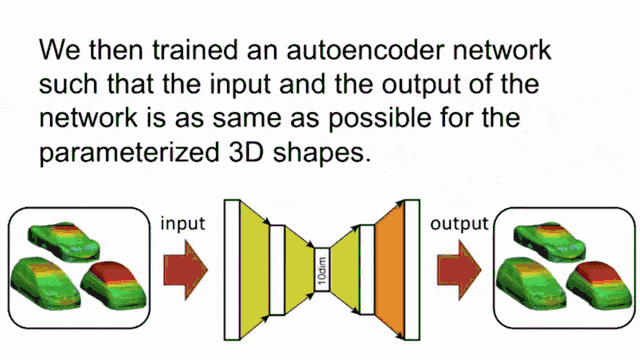An example for “auto”-encoding shapes (pun intended). Image created using gifify. Source: YouTube

So, here are our slides. Part two of our lecture and the topic autoencoders. Well, the concept of the autoencoder is that we want to use the ideas of feed-forward neural networks. You could say that a feed-forward neural network is a function of x that produces some encoding y.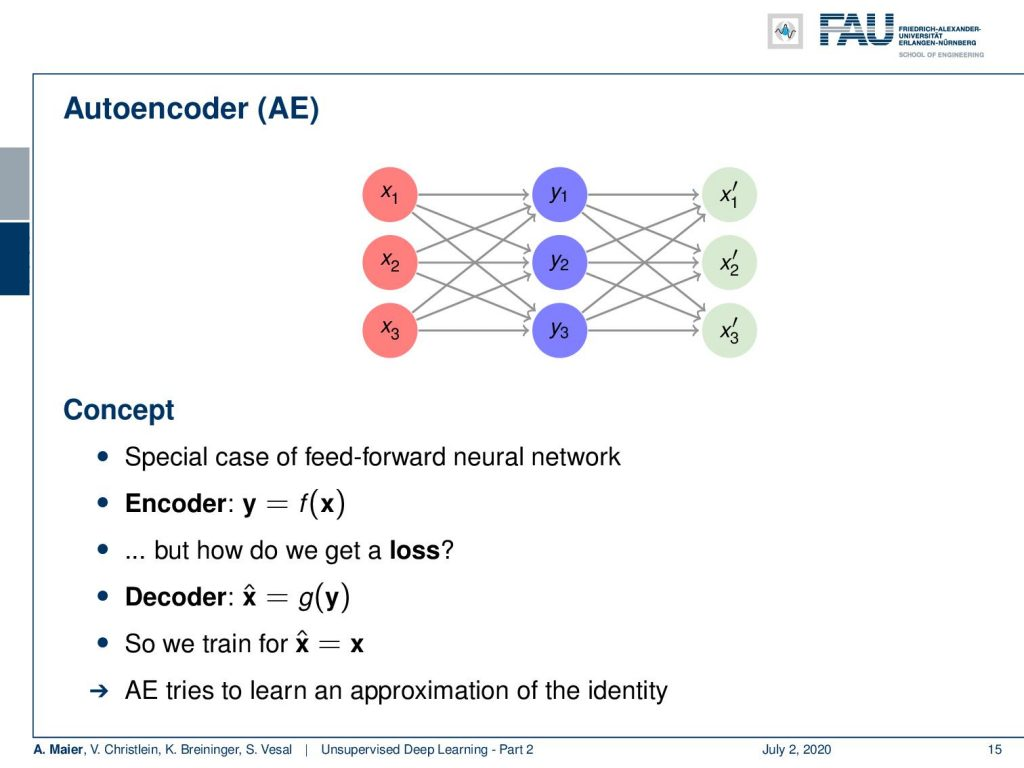Autoencoders try to reproduce the input given some additional constraints. Image under CC BY 4.0 from the Deep Learning Lecture.

Now, the problem is: “How can we generate a loss in such a constellation?” The idea is rather simple. We add an additional layer here on top and the purpose of the layer is to compute decoding. So, we have another layer that is g(y). g(y) produces some x hat. The loss that we can then define is that x hat and x need to be the same. So the autoencoder tries to learn an approximation of the identity. Well, that sounds rather simple. To be honest, if we have exactly the same number of nodes in the input and in the hidden layer for y here, then the easiest solution would probably be the identity. So why is this useful at all?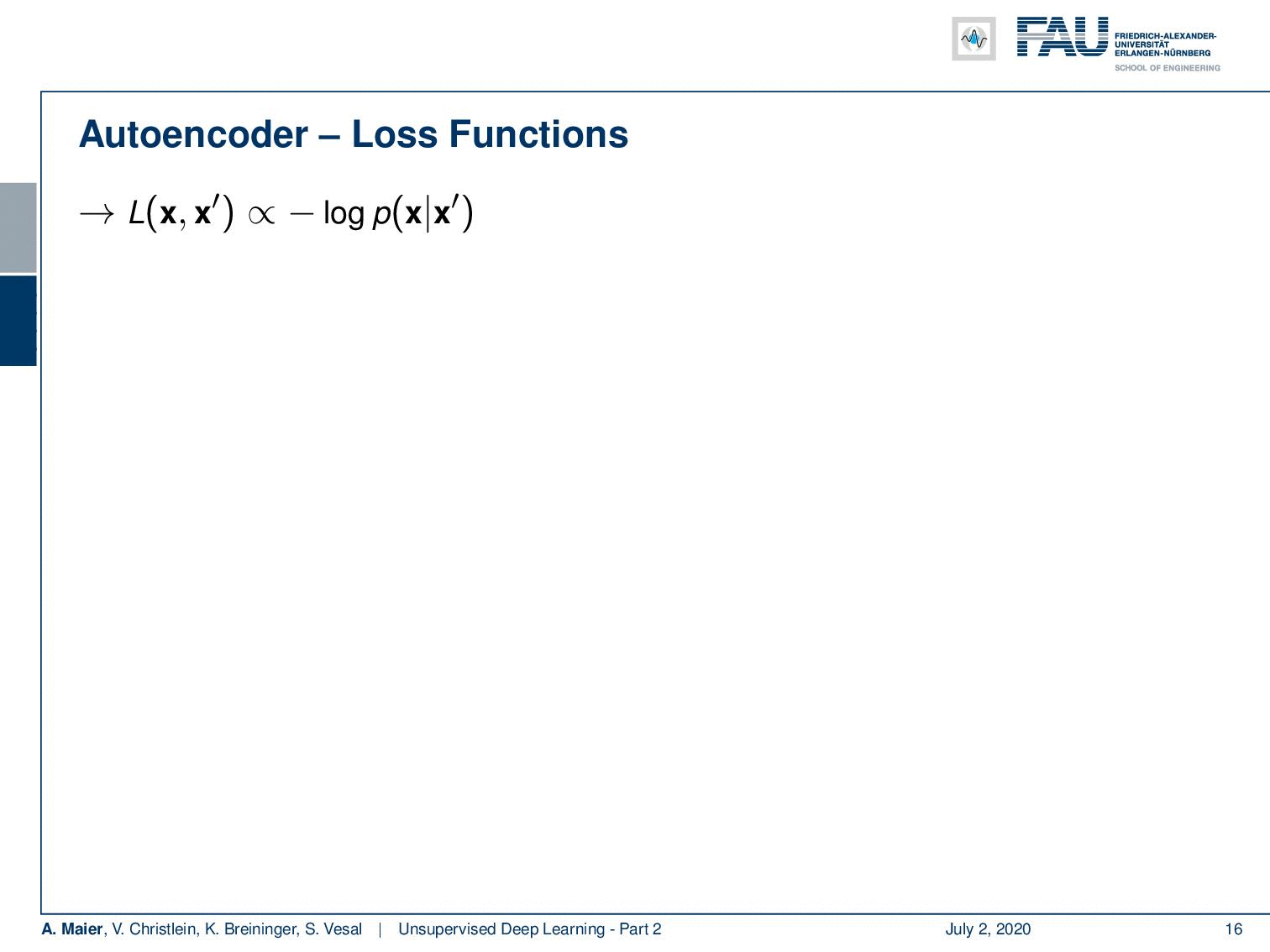Different autoencoder loss functions. Image under CC BY 4.0 from the Deep Learning Lecture.

Well, let’s look at some loss functions. What you can typically use is a loss function that then operates here on x and some x‘. It can be proportional to a negative log-likelihood function where you have p(x|x‘) and resulting functions. Then, in a similar way, as we’ve seen earlier in this class, you can use the squared L2 norm where you assume your probability density of a function to be a normal distribution with uniform variance. Then, you end up with the L2 loss. It is simply x minus x‘ and this encapsulated in an L2 norm. Of course, you can also do things like cross-entropy. So, if you assume the Bernoulli distribution, you see that we end up exactly with our cross-entropy. This is simply the sum over the weighted x times the logarithm of x‘ subscript i plus 1-x subscript i times the logarithm of 1 – x‘ subscript i. Remember that if you want to use it this way, then your x‘s need to be in the range of probabilities. So, if you want to apply this kind of loss function, then you may want to use it in combination with a softmax function.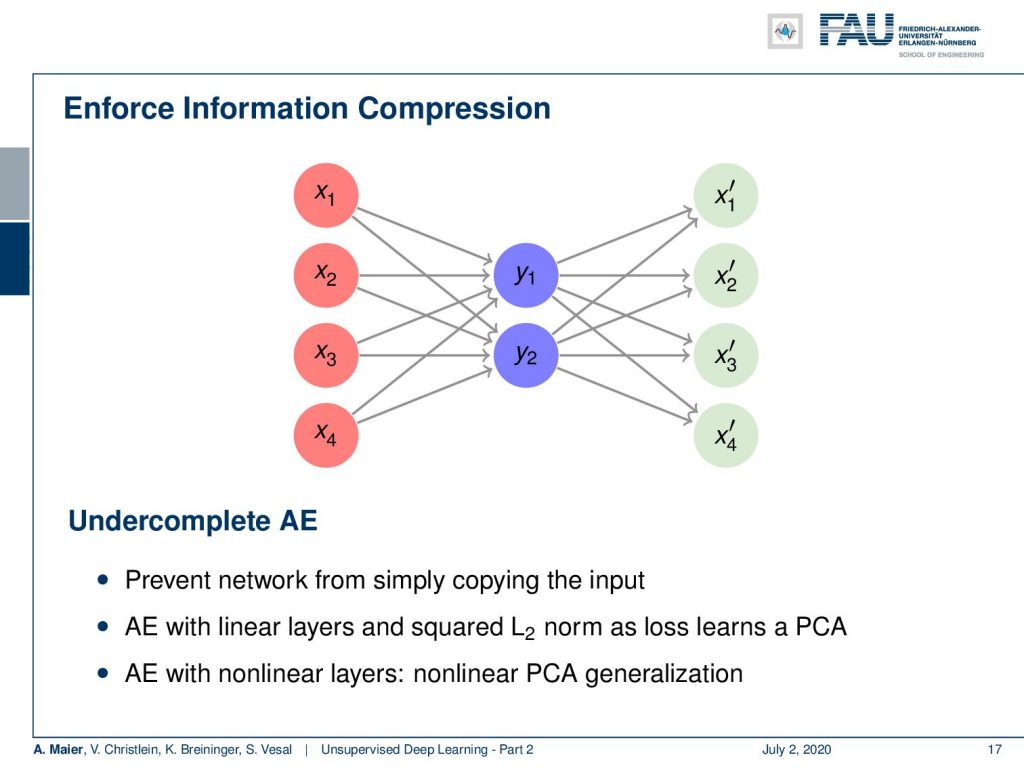The under complete autoencoder. Image under CC BY 4.0 from the Deep Learning Lecture.

Ok, so here are some typical strategies to construct such autoencoders. I think one of the most popular ones is the undercomplete autoencoder. So here, you enforce information compression by using fewer neurons in the hidden layer. You try to find a transform that does essentially a dimensionality reduction to the hidden layer. Then, you try to expand from this hidden layer onto the original data domain and try to find a solution that produces a minimum loss. So, you try to learn compression here. By the way, if you do this with linear layers and squared L2 norm, you essentially learn a principal component analysis (PCA). If you use it with nonlinear layers, you end up with something like a nonlinear PCA generalization.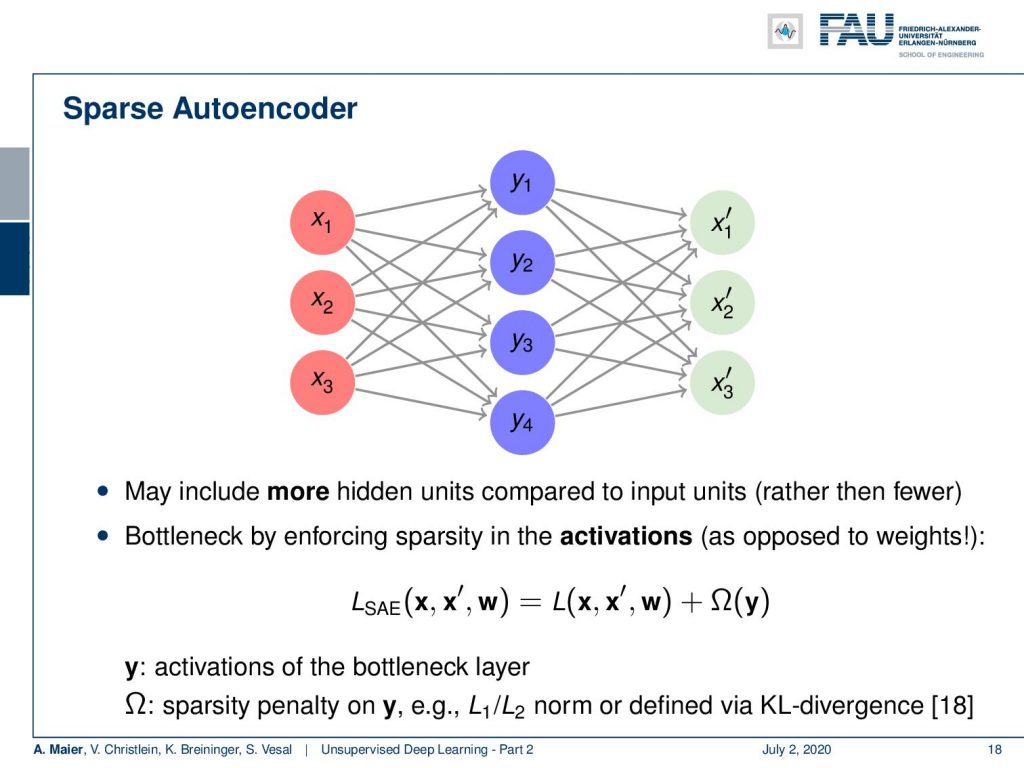The sparse autoencoder. Image under CC BY 4.0 from the Deep Learning Lecture.

There are also things like these sparse autoencoders. Here, we have a different idea. We even increase the number of neurons, to resemble a one-hot encoded vector. So, you may say: “Why would you increase the number of your neurons? Then, you could even find a much simpler solution like the identity and neglect a couple of those neurons!” So, this idea will not work straightforwardly. You have to enforce sparsity which is also coining the name sparse autoencoder. Here, you have to enforce sparsity in the activations using some additional regularization. For example, you can do this with an L1 norm on the activations in y. Remember, the sparsity in the sparse autoencoder stems from sparsity in the activations, not from sparsity in the weights. If you look at your identity, then you see this is simply a diagonal matrix with ones on the diagonal. So, this would also be a very sparse solution. So, again enforce the sparsity on the activations, not on the weights.

What else can be done? Well, you can use autoencoder variations. You can combine it essentially with all the recipes we’ve learned so far in this class. You can build convolutional autoencoders. There, you replace the fully connected layers with convolutional layers and you can optionally also add pooling layers.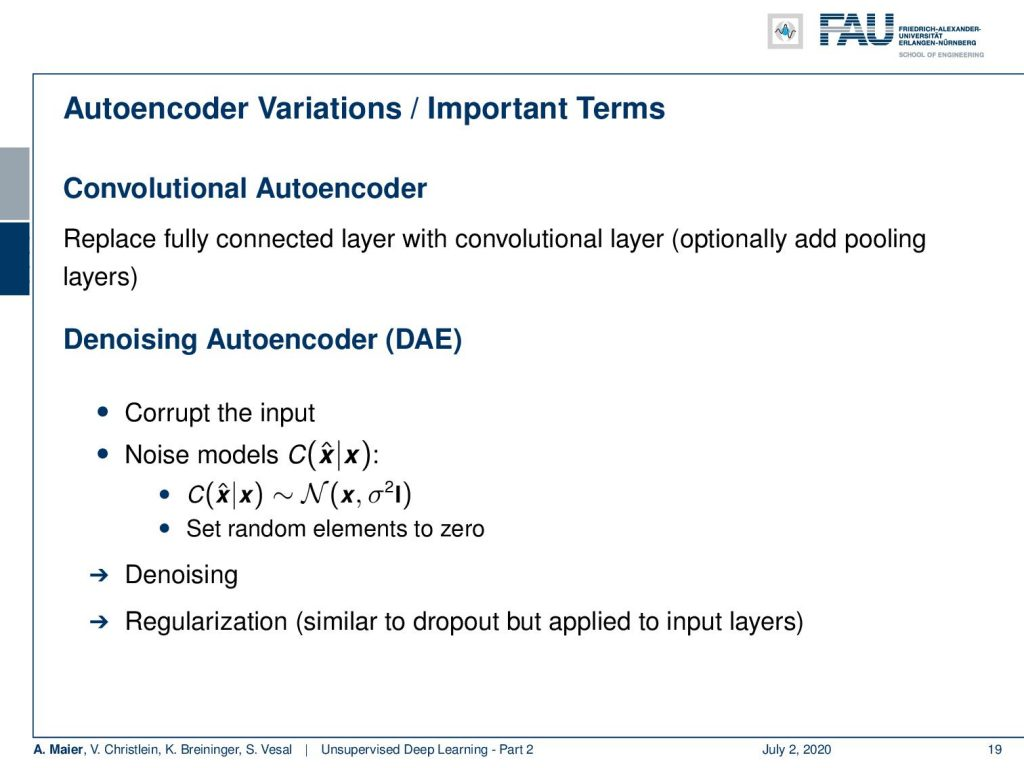More autoencoder variants. Image under CC BY 4.0 from the Deep Learning Lecture.

There is the denoising autoencoder which is also a very interesting concept. There you corrupt the input with noise and the target is then the noise-free original sample. So, this then results in a trained system that does not just do dimensionality reduction or finding a sparse representation. At the same time, it also performs denoising and you could argue that this is an additional regularization that is similar to dropout but essentially applied to the input layers. There’s also a very interesting paper called noise2noise where they show that you can even build such denoising auto-encoders even if you have a noisy target. The key here then is that you have a different noise pattern in the input and in the target and this way you can also train a denoising autoencoder at least if you build on top of convolutional autoencoders.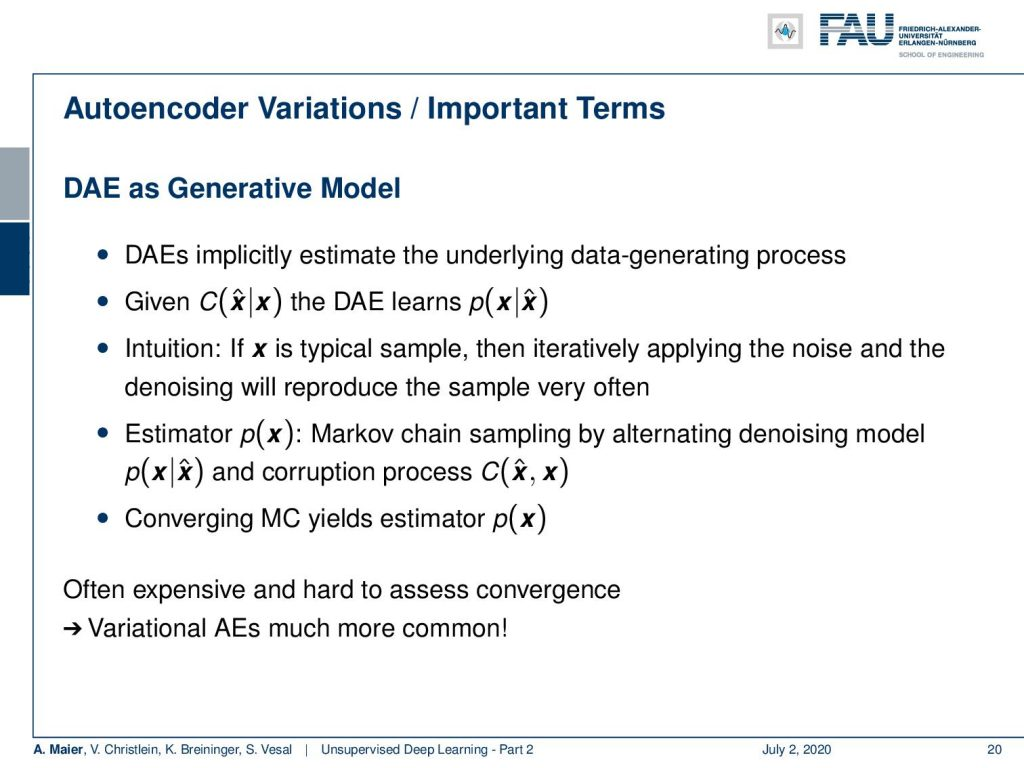Denoising autoencoders are also used for image generation. Image under CC BY 4.0 from the Deep Learning Lecture.

Now, you can even go as far as using the denoising autoencoder as a generative model. The idea here is that if you sample your corruption model, your noise model frequently. Then, the denoising autoencoder learns the probability of the respective input. So, if you have x as a typical sample, then by iteratively applying the noise and the denoising will reproduce this sample very often. So, you can then use a Markov chain and you alternate the denoising model and the corruption process. This then leads to an estimator of p(x). To be honest, this is often expensive and it’s very hard to assess the convergence which is the reason why so-called variational autoencoders are much more common. We will talk about those variational autoencoders in a couple of slides.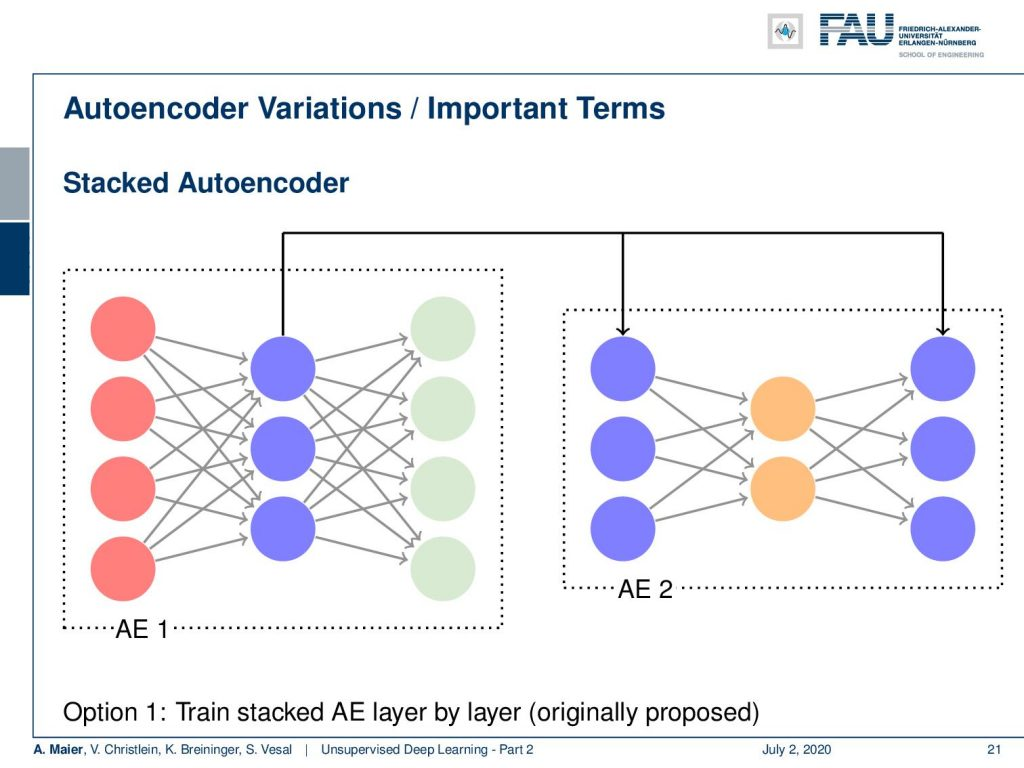Stacked autoencoders nest autoencoders. Image under CC BY 4.0 from the Deep Learning Lecture.

There are some variations that we need to introduce first. There’s the so-called stacked autoencoder. In the stacked autoencoder, you essentially put an autoencoder inside of an autoencoder. This allows us to build essentially deep autoencoders. We see this here in the concept. Autoencoder 1 is using in the hidden layer the Autoencoder 2 which is indicated by the blue nodes. You can stack those into a single autoencoder.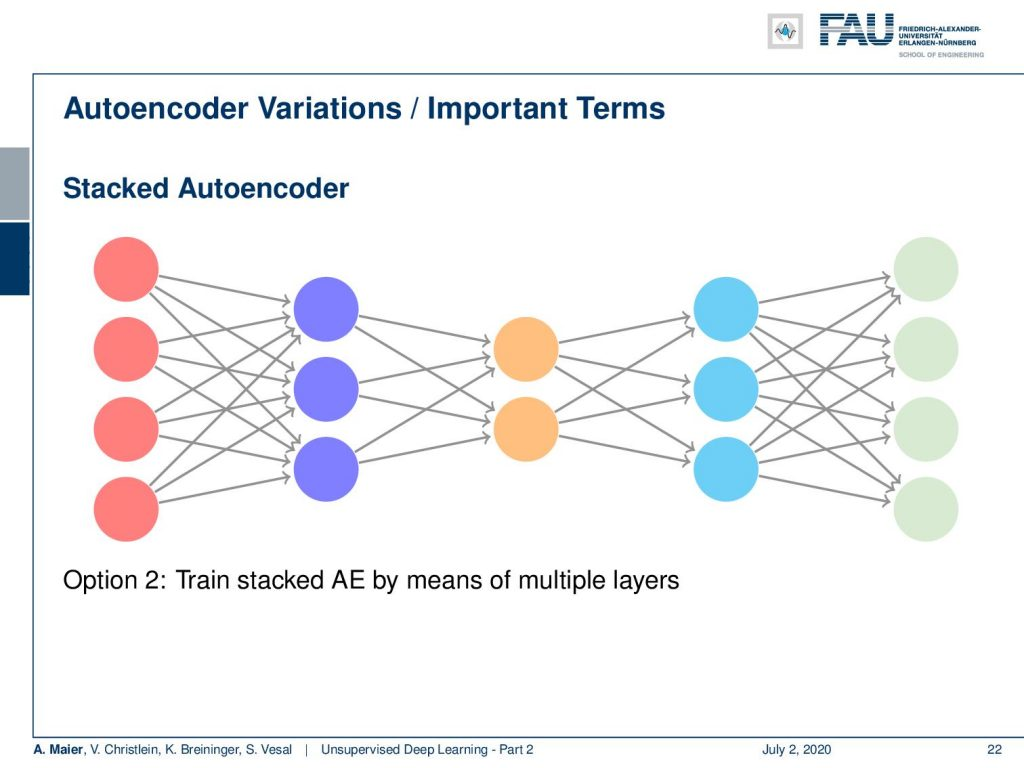The stacked version of this autoencoder. Image under CC BY 4.0 from the Deep Learning Lecture.

This gives rise to the following model and then the idea is to have a gradual dimensionality reduction. You already have guessed this: this is also something that is very commonly used together with convolutions and pooling.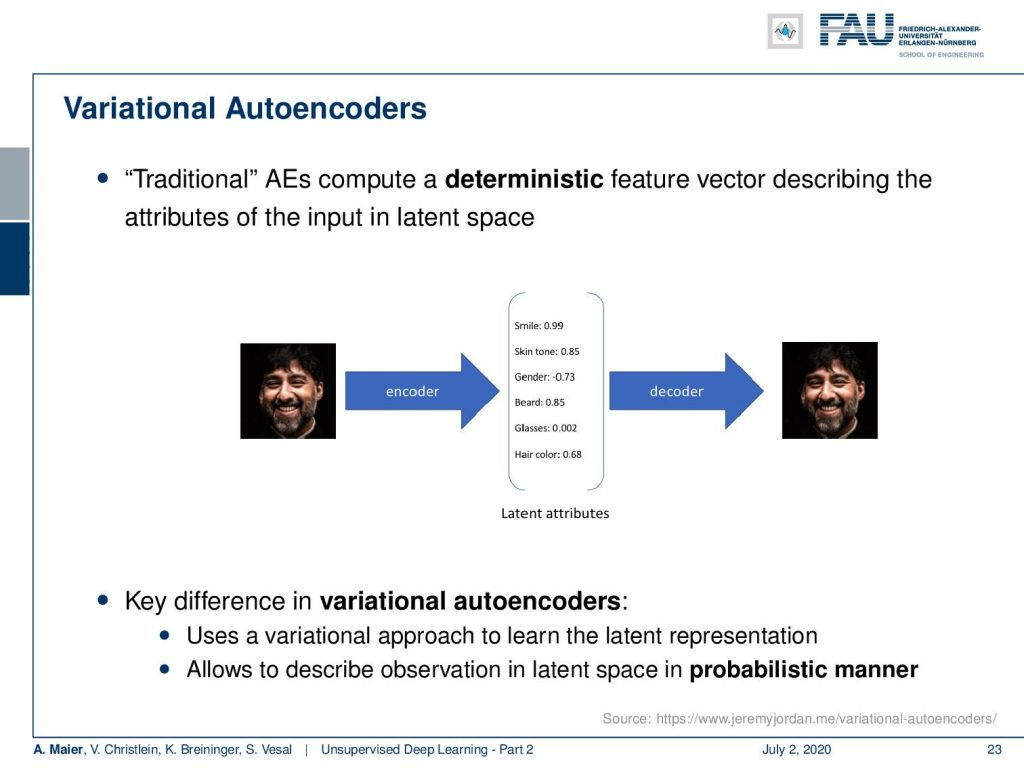Latent space interpretation in discrete space. Image under CC BY 4.0 from the Deep Learning Lecture.

Now let’s go into the concept of the variational autoencoders. This is a bit of a different concept. In the traditional autoencoders, you try to compute a deterministic feature vector that describes the attributes of the input in some kind of latent space. So let’s say, you had a latent space that characterizes different features, for example, of a face. Then, you could argue that this encoder then takes the image and projects it onto some probably unknown latent attributes. Here, we give them some additional interpretation. So for every attribute, you have a certain score and from the score then you generate the original image again. The key difference in variational auto-encoders is that you use a variational approach to learn the latent representation. It allows you to describe the latent space in a probabilistic manner.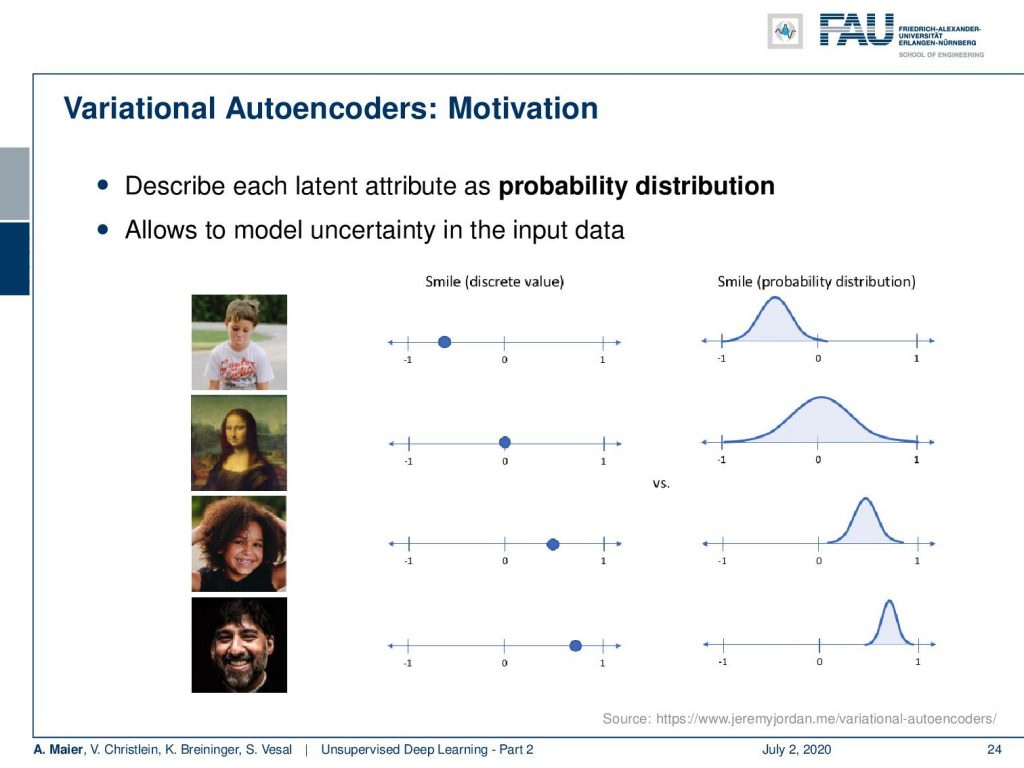Discrete vs. distributional modelling. Image under CC BY 4.0 from the Deep Learning Lecture.

So the idea is, it’s not just a simple category per dimension but instead you want to describe a probability distribution for each dimension. So, if we only had the regular autoencoders, you would see the scaling on the left-hand side here per image. The variable smile would be a discrete value, where you select one point where more or less smiling is available. In contrast, the variational autoencoder allows you to describe a probability distribution over the property “smile”. You can see here that in the first row there is not so much smiling. The second row – the Mona Lisa – you’re not sure whether she is smiling or not. People have been arguing over this for centuries. You can see that the variational autoencoder is able to describe this uncertainty by adding a variance over this latent variable. So, we can see we’re not sure whether this is a smile or not. Then, we have very clear instances of smiling, and then, of course, the distribution has a much lower standard deviation.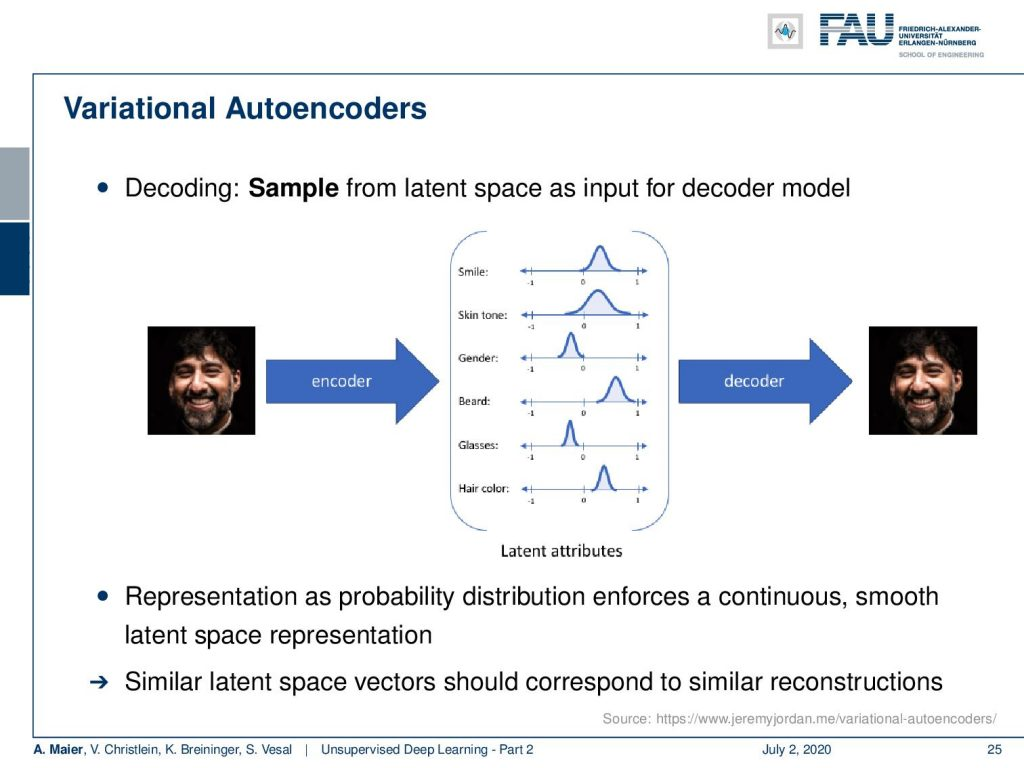Variational autoencoders model the latent space using distributions. Image under CC BY 4.0 from the Deep Learning Lecture.

So how would this work then? Well, you have some encoder that maps your input onto the latent attribute and the decoder is then sampling this distribution in order to produce the final output. So, this means that we have the representation as a probability distribution that enforces a continuous and smooth latent space representation. Similar latent space vectors should correspond to similar reconstructions.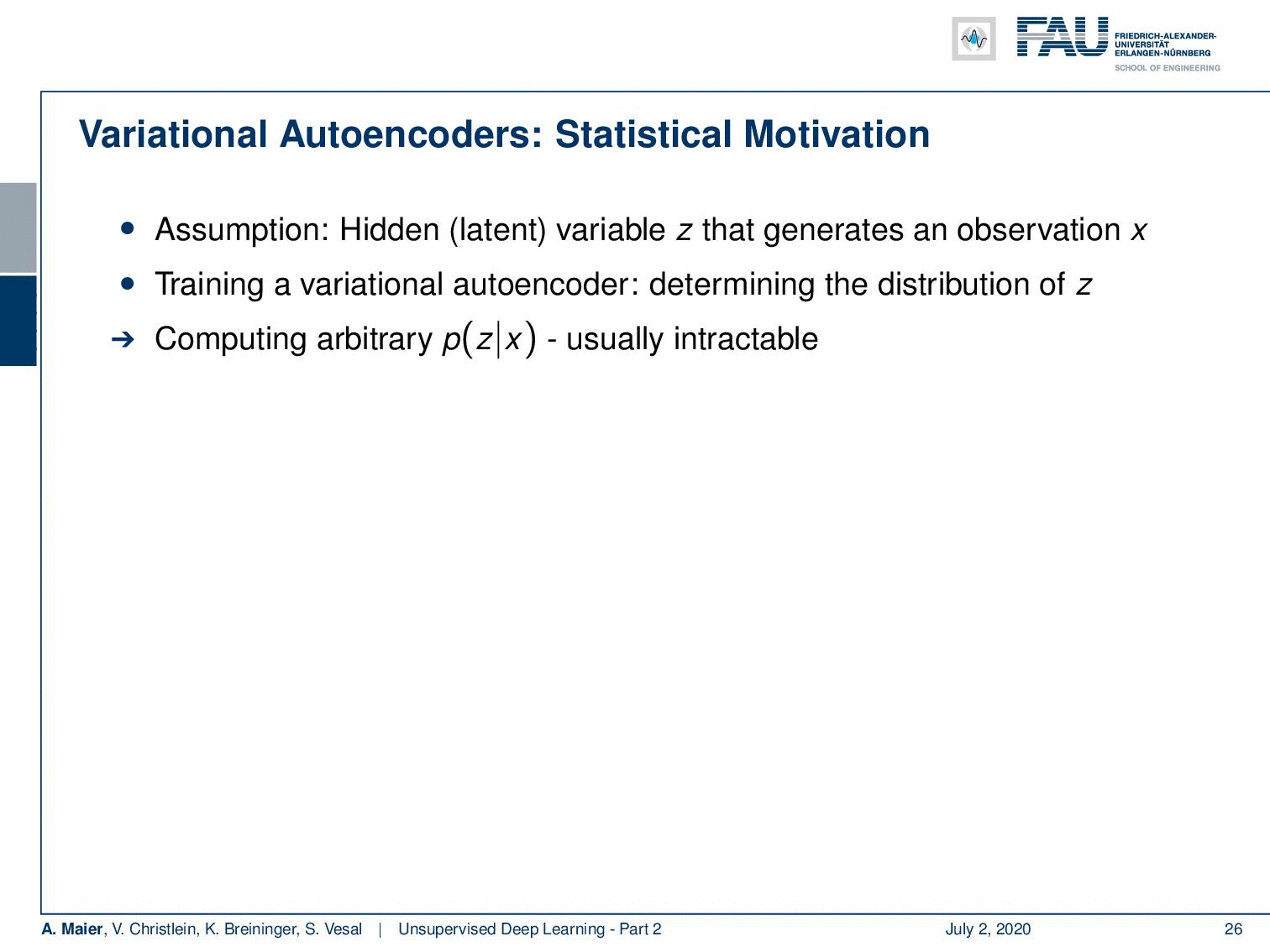Statistical motivation for variational autoencoders. Image under CC BY 4.0 from the Deep Learning Lecture.

Well, the assumption here is that we have some hidden latent variable z that generates some observation x. Then you can train the variational autoencoder by determining the distribution of z. Then, the problem is that the computation of this distribution p(z|x) is usually intractable. So, we somehow have to approximate our p(z|x) by a tractable distribution. This means that the tractable distribution q then leads to the problem that we have to determine the parameters of q. For example, you can use a Gaussian distribution for this. Then, you can use this to define Kullback-Leibler Divergence between P(z|x) and q(z|x). This is equivalent to maximizing the reconstruction likelihood of the expected value of the logarithm of p(x|z) minus the KL divergence between q(z|x) and p(z). This forces q(z|x) to be similar to the true prior distribution p(z).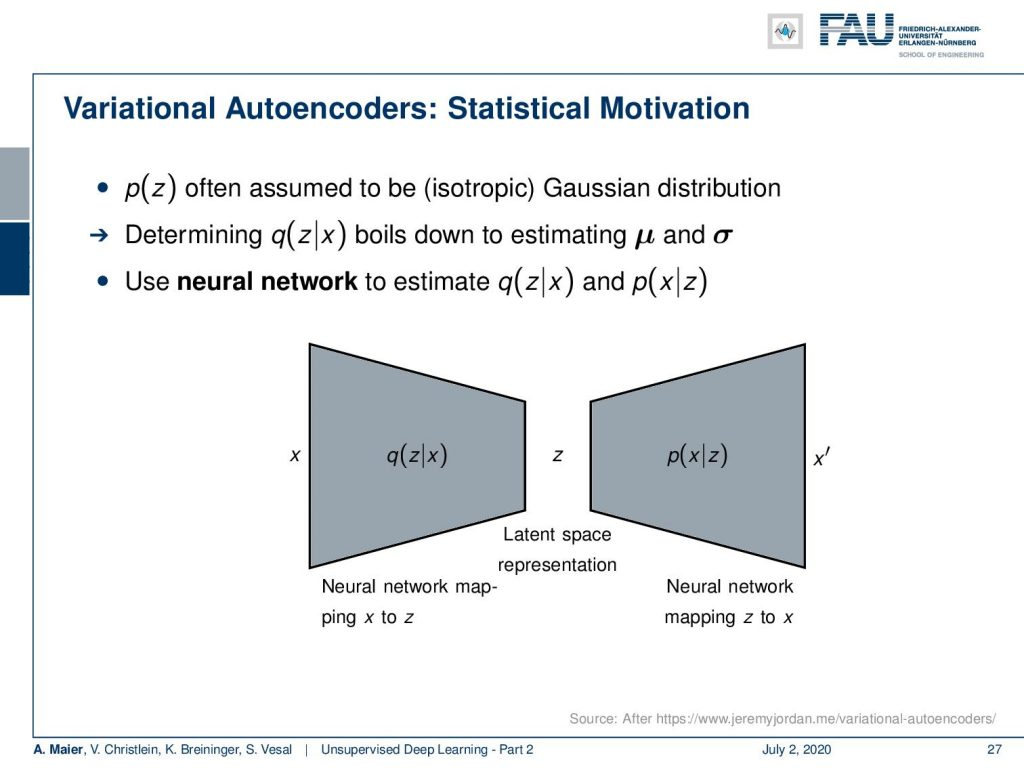Sketch of the variational autoencoder design. Image under CC BY 4.0 from the Deep Learning Lecture.

So now, p(z) is often assumed to be the isotropic Gaussian distribution. Determining now q(z|x) boils down to estimating the parameter vectors μ and σ. So, we do this using a neural network. Who might have guessed that? We estimate our q(z|x) and p(x|z). So here, you see the general outline. Again, you see this autoencoder structure and we have this encoder q(z|x) that produces the latent space representation and then our p(x|z) that produces again our output x‘. x‘ is supposed to be similar to x.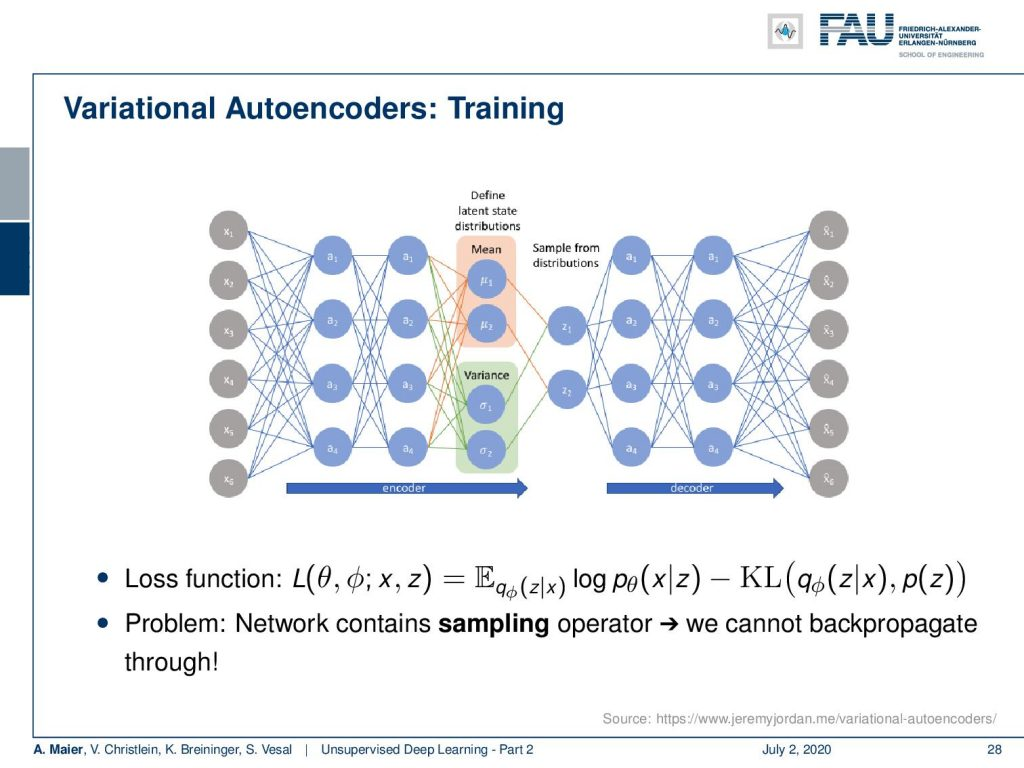Overview on the variational autoencoder network architecture. Image under CC BY 4.0 from the Deep Learning Lecture.

So, let’s look at this in some more detail. You can see that in the encoder branch, we essentially have a classic home feed-forward neural network that reduces the dimensionality. What we do in the following is we introduce one key change. This is indicated here in light red and light green. The key idea here now is that in the specific layer that is indicated by the colors, we change the interpretation of the activations. In those neurons, they’re essentially just feed-forward layers, but we interpret the top two neurons here as means of our latent distribution and the bottom two neurons as variances of the latent distributions. Now, the key problem is that in order to go to the next layer, we have to sample from those distributions in order to get the actual observations and perform the decoding on them. So how can this potentially be done? Well, we have a problem here because we cannot backpropagate through the random sampling process.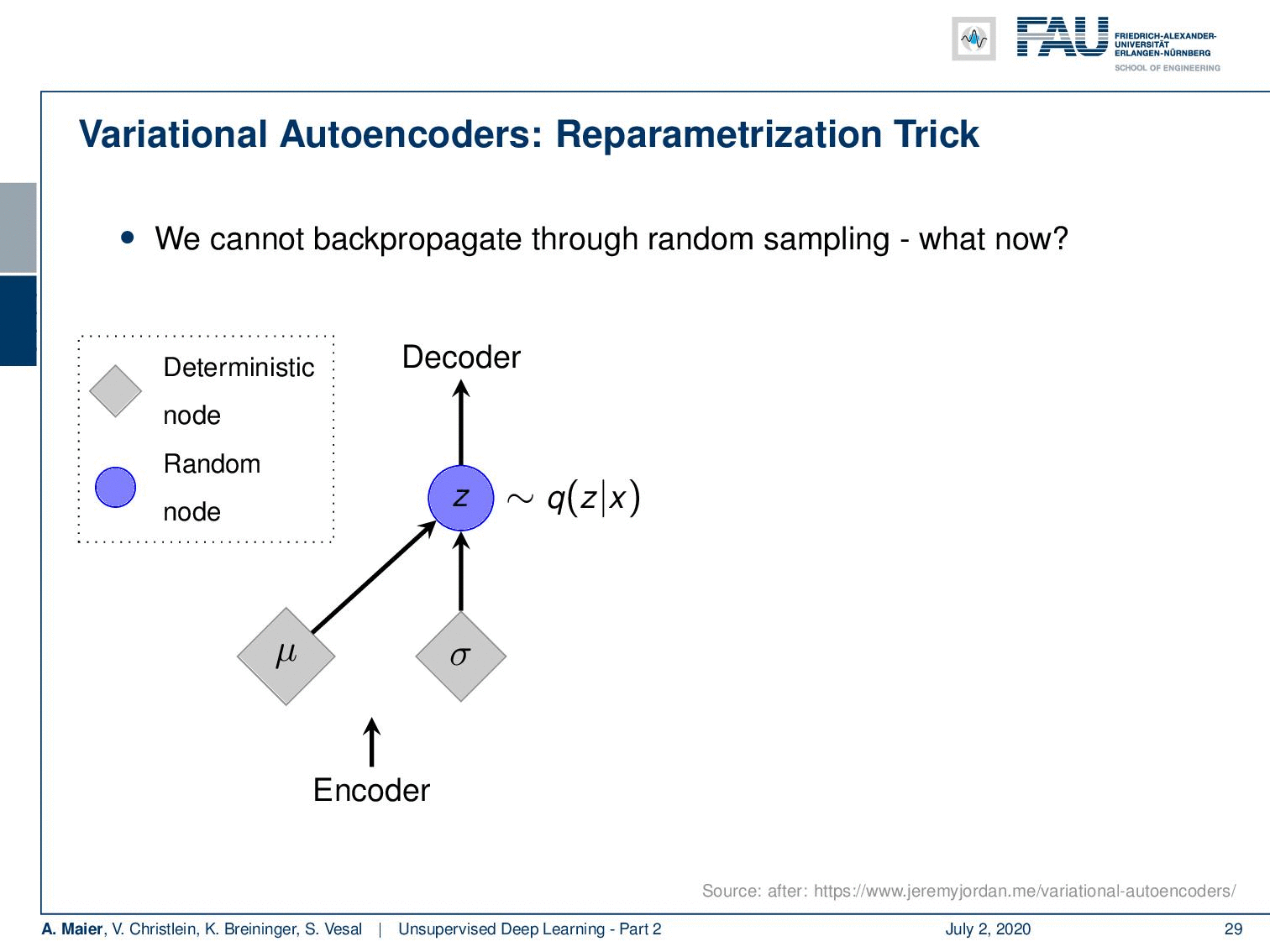The reparametrization trick. Image under CC BY 4.0 from the Deep Learning Lecture.

So the idea here is that the encoder produces the means and variances. Then, we produce some z that is a sampling of our function q(z|x). So, this is a random node and the randomness has the problem that we don’t know how to backpropagate for this node. So, the key element that we introduced in the forward-pass here is that we reparameterize z. z is determined deterministically as the μ plus σ times some ε. ε is the random information. It now stems from a random generator that is simply connected to z. For example, you can choose it to be a Gaussian distribution with zero mean and unit variance. So, this then allows us to backpropagate into μ and σ because the random node is here on the right-hand side and we don’t need to backpropagate into the random node. So, by this reparametrization trick, we can even introduce sampling into a network as a layer. So, this is pretty exciting and as you have already guessed. this is also very nice because we can then use this noise in order to generate random samples from this specific distribution. So, we can use the right-hand side of our autoencoder in order to produce new samples. All of this is deterministic in the backpropagation and you can compute your gradients in the same way as we used to. You can apply your backpropagation algorithm as before.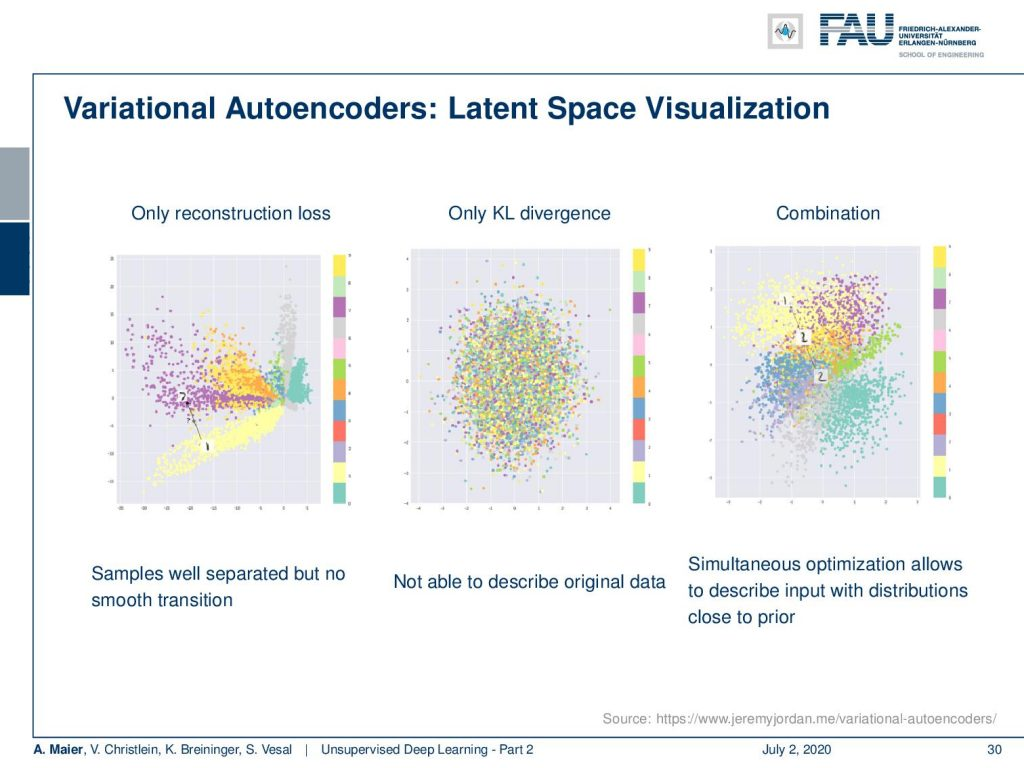Comparison of different latent spaces. Image under CC BY 4.0 from the Deep Learning Lecture.

So what effect does this have? Well here, we have some latent space visualizations. If you use only the reconstruction loss, you can see that samples are well separated but there is no smooth transition. Obviously, if you only had the KL divergence then you would not be able to describe the original data. So, you need the combination where you’re simultaneously optimizing with respect to the input distributions and the respective KL divergence. So, this is pretty cool because then we can generate new data by sampling from the distributions in the latent space and then you reconstruct with the decoder the diagonal prior enforces independent latent variables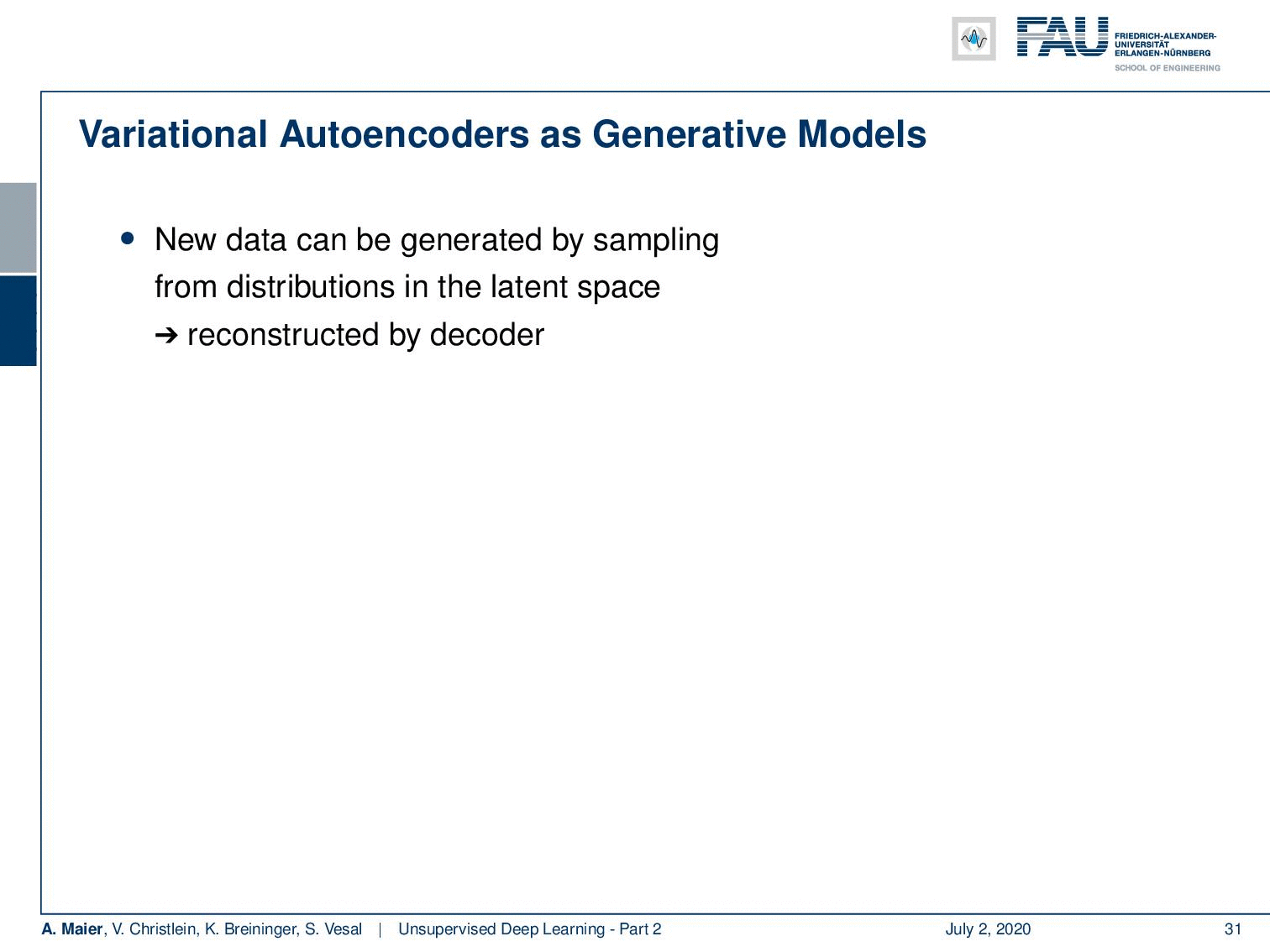Image generation using variational autoencoders. Image under CC BY 4.0 from the Deep Learning Lecture.

So, we can encode different factors of variation. Here, we have an example where we smoothly vary the degree of smiling and the head pose. You can see that this kind of disentangling actually works.

So, let’s summarize. The variational autoencoder is a probabilistic model that allows you to generate data from an intractable density. We are able to optimize a variational lower bound instead and this is trained by backpropagation using reparametrization.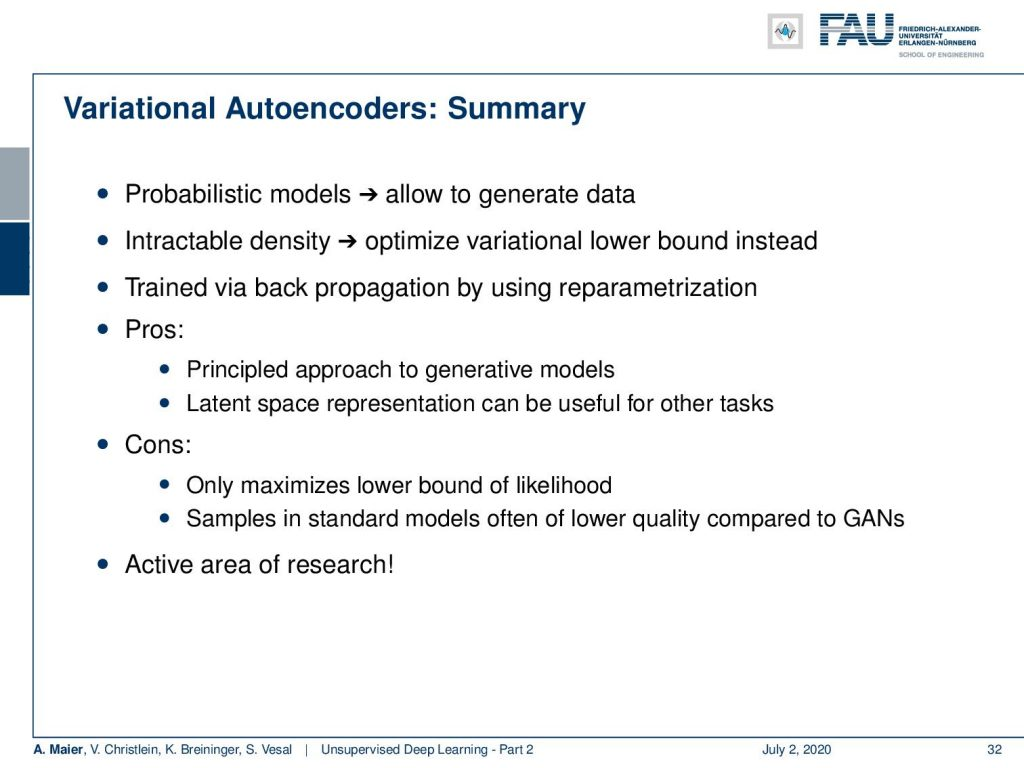Summary of variational autoencoders. Image under CC BY 4.0 from the Deep Learning Lecture.

The pros are that we have a principled approach to generative modeling. The latent space representation can be very useful for other tasks but the cons are that this only maximizes a lower bound of likelihood. So, the samples in standard models are often of lower quality compared to generative adversarial networks. This is still an active area of research. So, in this video, I gave you a very nice wrap up of autoencoders and the important techniques are, of course, the simple autoencoder, the undercomplete autoencoder, the sparse autoencoder, and the stacked autoencoder. Finally, we’ve been looking into variational autoencoders in order to be able to also generate data and in order to be able to describe probability distributions in our latent variable space.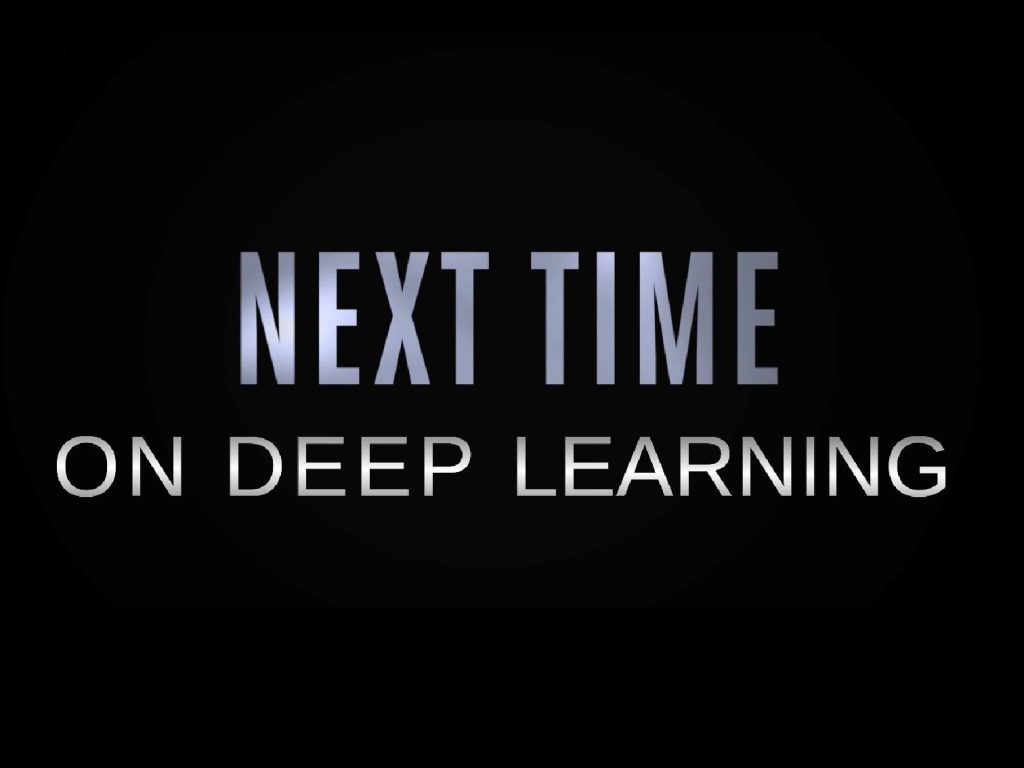More exciting things coming up in this deep learning lecture. Image under CC BY 4.0 from the Deep Learning Lecture.

In the next video, we will see that data generation is a very interesting task and we can essentially do it from unsupervised data. This is why we will look into the generative adversarial networks. You will see that this is a very interesting technique that has led to widespread use in many different applications in order to use the power of deep neural networks to perform data generation. So, I hope you liked this video. We discussed very important points of unsupervised learning and I think these are state-of-the-art techniques that are widely used. So, if you like this video, please stay tuned and looking forward to meeting you in the next one. Thank you very much. Goodbye!

If you liked this post, you can find more essays here, more educational material on Machine Learning here, or have a look at our Deep LearningLecture. I would also appreciate a follow on YouTubeTwitterFacebook, or LinkedIn in case you want to be informed about more essays, videos, and research in the future. This article is released under the Creative Commons 4.0 Attribution License and can be reprinted and modified if referenced. If you are interested in generating transcripts from video lectures try AutoBlog.

Link – Variational Autoencoders:
Link – NIPS 2016 GAN Tutorial of Goodfellow
Link – How to train a GAN? Tips and tricks to make GANs work (careful, not
everything is true anymore!)
Link - Ever wondered about how to name your GAN?

## References

 Xi Chen, Xi Chen, Yan Duan, et al. “InfoGAN: Interpretable Representation Learning by Information Maximizing Generative Adversarial Nets”. In: Advances in Neural Information Processing Systems 29. Curran Associates, Inc., 2016, pp. 2172–2180.
 Pascal Vincent, Hugo Larochelle, Isabelle Lajoie, et al. “Stacked denoising autoencoders: Learning useful representations in a deep network with a local denoising criterion”. In: Journal of Machine Learning Research 11.Dec (2010), pp. 3371–3408.
 Emily L. Denton, Soumith Chintala, Arthur Szlam, et al. “Deep Generative Image Models using a Laplacian Pyramid of Adversarial Networks”. In: CoRR abs/1506.05751 (2015). arXiv: 1506.05751.
 Richard O. Duda, Peter E. Hart, and David G. Stork. Pattern classification. 2nd ed. New York: Wiley-Interscience, Nov. 2000.
 Asja Fischer and Christian Igel. “Training restricted Boltzmann machines: An introduction”. In: Pattern Recognition 47.1 (2014), pp. 25–39.
 John Gauthier. Conditional generative adversarial networks for face generation. Mar. 17, 2015. URL: http://www.foldl.me/2015/conditional-gans-face-generation/ (visited on 01/22/2018).
 Ian Goodfellow. NIPS 2016 Tutorial: Generative Adversarial Networks. 2016. eprint: arXiv:1701.00160.
 Martin Heusel, Hubert Ramsauer, Thomas Unterthiner, et al. “GANs Trained by a Two Time-Scale Update Rule Converge to a Local Nash Equilibrium”. In: Advances in Neural Information Processing Systems 30. Curran Associates, Inc., 2017, pp. 6626–6637.
 Geoffrey E Hinton and Ruslan R Salakhutdinov. “Reducing the dimensionality of data with neural networks.” In: Science 313.5786 (July 2006), pp. 504–507. arXiv: 20.
 Geoffrey E. Hinton. “A Practical Guide to Training Restricted Boltzmann Machines”. In: Neural Networks: Tricks of the Trade: Second Edition. Berlin, Heidelberg: Springer Berlin Heidelberg, 2012, pp. 599–619.
 Phillip Isola, Jun-Yan Zhu, Tinghui Zhou, et al. “Image-to-Image Translation with Conditional Adversarial Networks”. In: (2016). eprint: arXiv:1611.07004.
 Diederik P Kingma and Max Welling. “Auto-Encoding Variational Bayes”. In: arXiv e-prints, arXiv:1312.6114 (Dec. 2013), arXiv:1312.6114. arXiv: 1312.6114 [stat.ML].
 Jonathan Masci, Ueli Meier, Dan Ciresan, et al. “Stacked Convolutional Auto-Encoders for Hierarchical Feature Extraction”. In: Artificial Neural Networks and Machine Learning – ICANN 2011. Berlin, Heidelberg: Springer Berlin Heidelberg, 2011, pp. 52–59.
 Luke Metz, Ben Poole, David Pfau, et al. “Unrolled Generative Adversarial Networks”. In: International Conference on Learning Representations. Apr. 2017. eprint: arXiv:1611.02163.
 Mehdi Mirza and Simon Osindero. “Conditional Generative Adversarial Nets”. In: CoRR abs/1411.1784 (2014). arXiv: 1411.1784.
 Alec Radford, Luke Metz, and Soumith Chintala. Unsupervised Representation Learning with Deep Convolutional Generative Adversarial 2015. eprint: arXiv:1511.06434.
 Tim Salimans, Ian Goodfellow, Wojciech Zaremba, et al. “Improved Techniques for Training GANs”. In: Advances in Neural Information Processing Systems 29. Curran Associates, Inc., 2016, pp. 2234–2242.
 Andrew Ng. “CS294A Lecture notes”. In: 2011.
 Han Zhang, Tao Xu, Hongsheng Li, et al. “StackGAN: Text to Photo-realistic Image Synthesis with Stacked Generative Adversarial Networks”. In: CoRR abs/1612.03242 (2016). arXiv: 1612.03242.
 Han Zhang, Tao Xu, Hongsheng Li, et al. “Stackgan: Text to photo-realistic image synthesis with stacked generative adversarial networks”. In: arXiv preprint arXiv:1612.03242 (2016).
 Bolei Zhou, Aditya Khosla, Agata Lapedriza, et al. “Learning Deep Features for Discriminative Localization”. In: 2016 IEEE Conference on Computer Vision and Pattern Recognition (CVPR). Las Vegas, June 2016, pp. 2921–2929. arXiv: 1512.04150.
 Jun-Yan Zhu, Taesung Park, Phillip Isola, et al. “Unpaired Image-to-Image Translation using Cycle-Consistent Adversarial Networks”. In: CoRR abs/1703.10593 (2017). arXiv: 1703.10593.